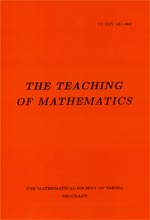﻿ The Teaching of Mathematics - Instructions for Authors ﻿
 THE TEACHING OF MATHEMATICSA case study of a student who created problems for a mathematics competition Andreas Poulos AbstractIn this article we present the conclusions drawn from our research on the subject of problem posing for mathematics competitions. We present the case study of a student who is familiar with problem solving, has understood what problem posing means and what is a mathematical problematic situation, has proven his mathematical skills, and is asked to create his own mathematical problem. The conclusions drawn from this research are, firstly, on the relation of mathematical creativity and mathematical problem posing, secondly, on the relation of mathematical education and self-education, and thirdly on the relationship between the creator of a problem and the potential solver of the problem.Keywords: Problem solving; problem posing; mathematical creativity; problem situation. Pages:  109$-$116 Volume  XXIII ,  Issue  2 ,  2020

﻿